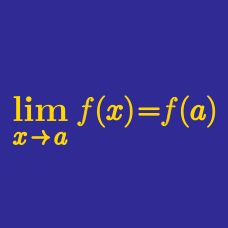Calculus

# Properties of Continuous Functions Warmup

The function $f,$ some of whose values are shown below, is a continuous function defined for all real numbers.

$\begin{array} {c|c|c|c|c|c|c|c} x & 0 & 1 & 2 & 3 & 4 \\ f(x) & 10 & 17 & 8 & -11 & -10 \\ \end{array}$

Between which two $x-$values does the intermediate value theorem guarantee a solution to $f(x) = 0?$Dylan goes on a 5-hour hike in the mountains. Let $f(t)$ represent Dylan's altitude at time $t$ hours after starting.

True or False? There is a maximum altitude in the set of altitudes reached by Dylan during the interval $[0,5].$Mehmet leaves home on an epic journey. During his travels, he visits valleys much lower than his home and mountaintops much higher than his home.

True or False: Mehmet must have visited at least one place that has the same altitude as his home.Mehmet leaves home on an epic journey. He is always aware of the price of an apple at the nearest vendor. He visits places where apples are much cheaper than they are at home, and places where apples are much more expensive than at home.

True or False: Mehmet must have visited at least one place outside of his local apple vendor's neighborhood where apples are the same price as they are at home.

On which interval(s) does the function $f(x) = x^2$ attain a maximum value?

$A = [1,2] = \{x | 1 \leq x \leq 2 \} \,\,\,\,\,\,$

$B = [1,2) = \{x | 1 \leq x < 2 \} \,\,\,\,\,\,$

(The number 2 is in $A$ but not in $B.)$

×